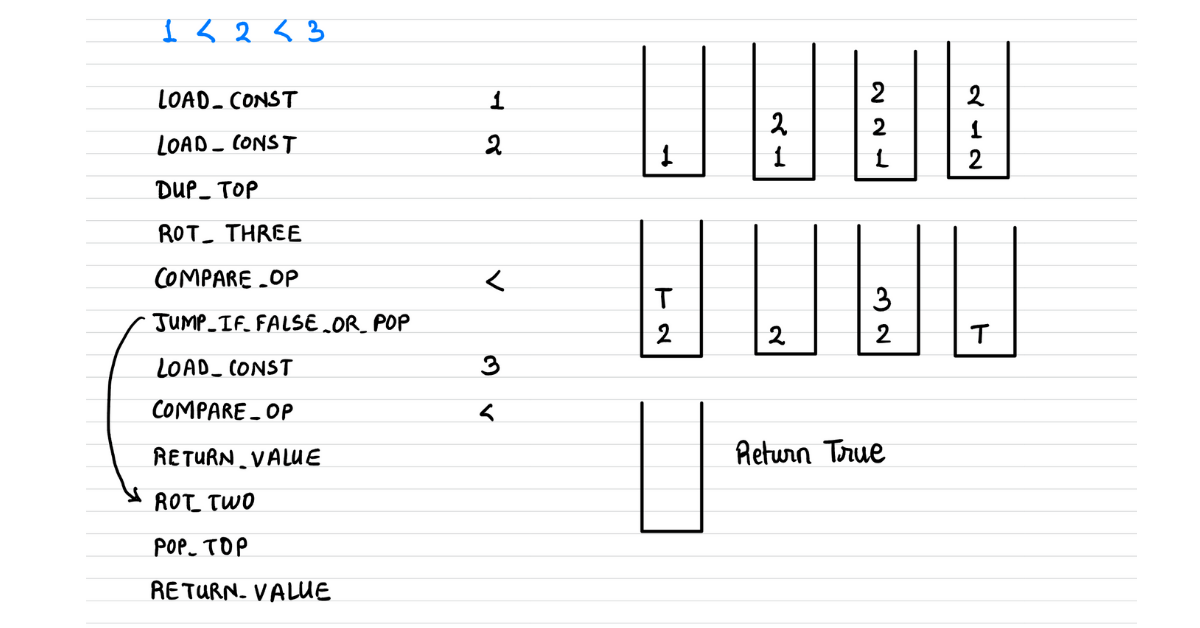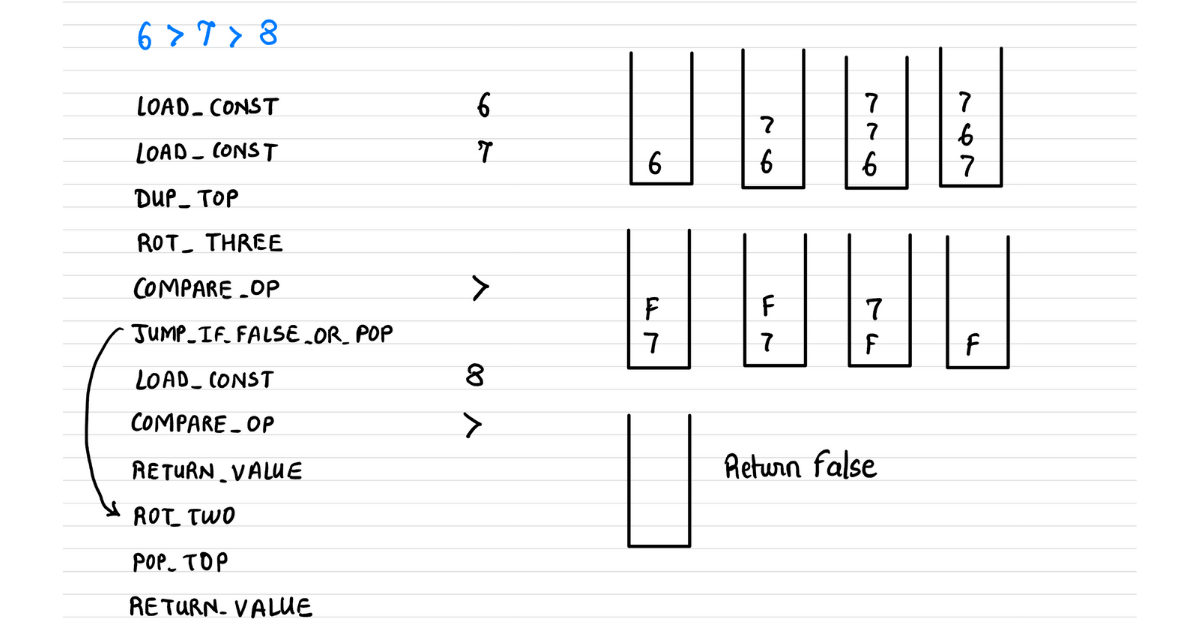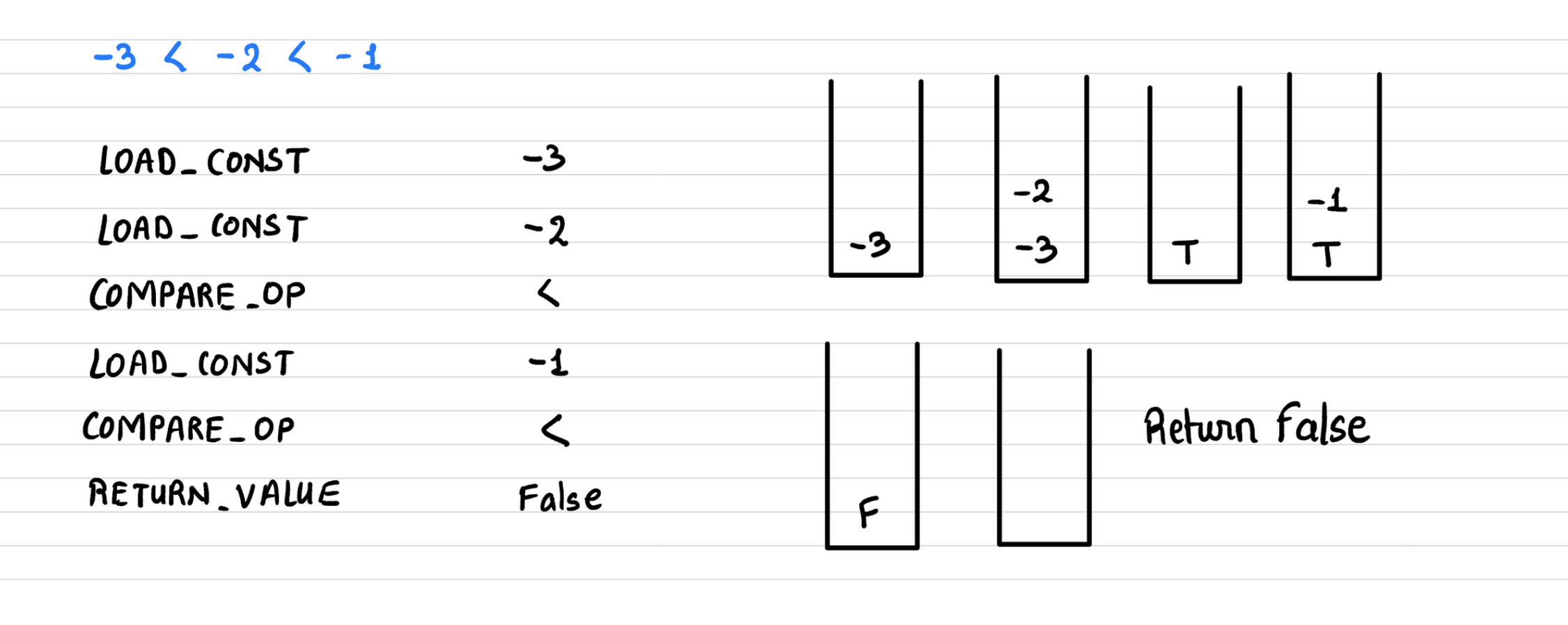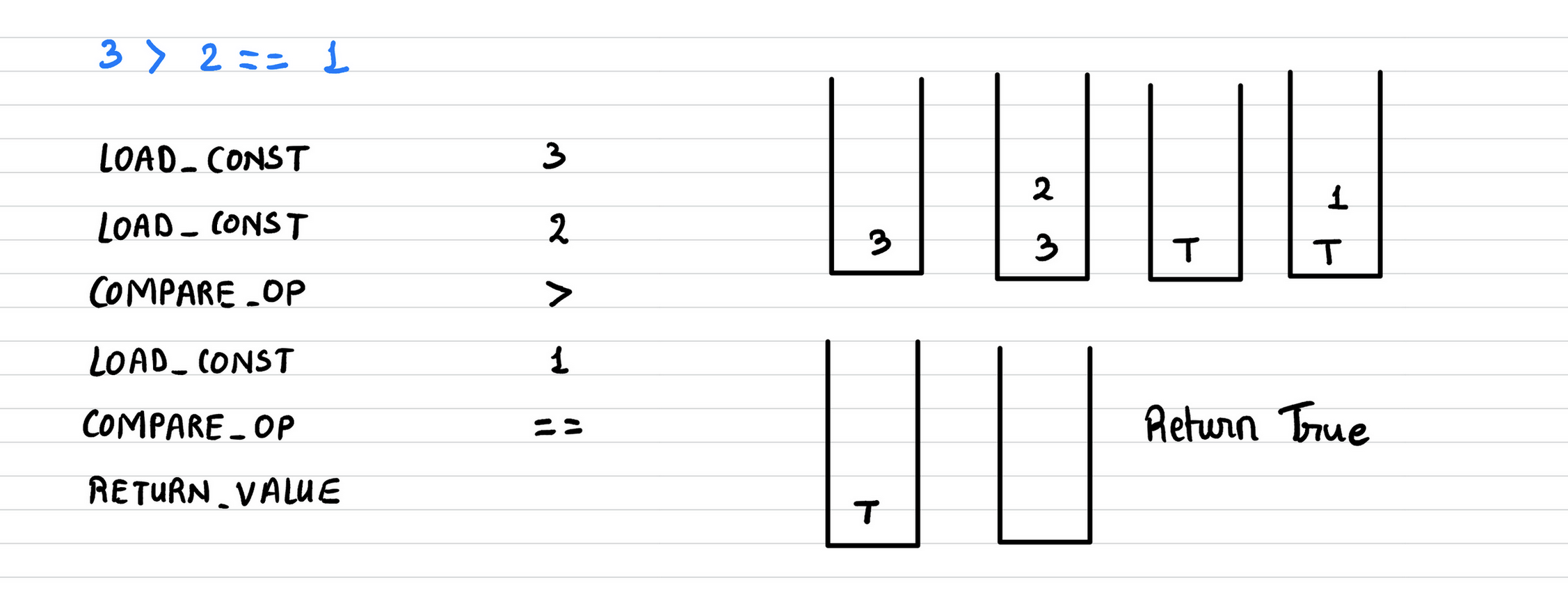# How Python evaluates chained comparison operators

Python supports chaining of comparison operators, which means if we wanted to find out if `b` lies between `a` and `c` we can do `a < b < c`, making code super-intuitive. Python evaluates such expressions like how we do in mathematics. which means `a < b < c` is evaluated as `(a < b) and (b < c)`. C language on the other hand, evaluates `a < b < c` as `((a < b) < c)`.

Depending on how we evaluate such expressions, the final evaluated changes. So, in python, if we evaluate `-3 < -2 < -1`, we get `True` and if evaluate `3 > 2 == 1` we get `False`.

``````>>> -3 < -2 < -1
True
>>> 3 > 2 == 1
False``````

But on the other hand, if we evaluate this very expression in C language the output is `False`.

``````#include <stdio.h>

int main(int argc, char *argv[]) {
printf("%d\n", -3 < -2 < -1);
printf("%d\n", 3 > 2 == 1);
return 0;
}

\$ gcc test.cpp
\$ ./a.out
0
1``````

It does so because `(-3 < -2) = True = 1` and `1 < -1 is False`. Also, to get a better understanding of how such expressions evaluate, try playing around with different values and see if your predicted value matches the actual output.

This essay is going to be extra special; in this one, we find out

• how Python evaluates chained comparison operators?
• how Python implements short-circuiting?
• how could you make Python-like evaluation a C-like evaluation? implying at the end of this essay we alter the CPython source code such that the expression `-3 < -2 < -1` will evaluate to `False`.

I know this sounds tempting, so let’s jump right into it.

# Chaining comparison operators

Python has a plethora of comparison operators like `<`, `>`, `<=`, `>=`, `==`, `!=`, `in`, and `is`. The output of the comparison operator is a boolean value - `True` or `False`. Python allows chaining of comparison operators which means, to check if `b` lies between `a` and `c`, we can simply do

``>>> a < b < c``

This is possible because internally Python evaluates this chained expression `a < b < c` as `(a < b) and (b < c)`. To make this efficient, the sub-expression `b` is evaluated only once and the evaluation also follows short-circuit evaluation; which means, if `(a < b)` is evaluated as `False` then Python would not evaluate further sub-expressions - `c` and `(b < c)`. Now that we have set the context, let’s find out what happens under the hood.

# Chaining under the hood

Disassembling the expression would give out the set of Python instructions that will be executed on the runtime’s execution stack. If we disassemble `a < b < c`, we get the following set of instructions. By the way, this is a great way to jump into the internals of anything in Python.

``````>>> import dis
>>> dis.dis('a < b < c')

4 DUP_TOP
6 ROT_THREE
8 COMPARE_OP               0 (<)
10 JUMP_IF_FALSE_OR_POP    18
14 COMPARE_OP               0 (<)
16 RETURN_VALUE
>>   18 ROT_TWO
20 POP_TOP
22 RETURN_VALUE``````

Here is the summary of what each of the above instructions does; having this understanding will help us understand the entire execution process.

• `LOAD_NAME`: Loads the variable on the top of the stack
• `DUP_TOP`: Duplicates the top of the stack
• `ROT_THREE`: Rotates the top 3 elements of the stack by 1, such that the second element becomes the top, the third becomes the second while the top becomes the third.
• `COMPARE_OP`: Pops the top two elements from the stack, compare them (depending on the operator), compute the output, and puts it on the top of the stack.
• `JUMP_IF_FALSE_OR_POP`: Checks if the top of the stack is `False` if it is false then jumps to provided offset, and if it is `True` it pops the value.
• `RETURN_VALUE`: Pops the top of the stack and returns it
• `ROT_TWO`: Rotates the top two elements of the stack such that the top elements become the second, while the second becomes the top.
• `POP_TOP`: Pops the top element from the stack, kind of discarding it.

You can find details about these opcodes in the file ceval.c. Now, let’s do an instruction by instruction walkthrough for the expression `1 < 2 < 3` to see how it evaluates to `True`.

## Evaluating `1 < 2 < 3`

When we run disassembler on `1 < 2 < 3` we get a similar disassembled code. It starts with the loading of two constant values `1` and `2` on the stack. Then it duplicates the top which makes our stack `2, 2, 1`. Now upon `ROT_THREE` the `2` on the top of the stack goes at the third spot while the other moves up one place. at this instruction, our stack looks like `2, 1, 2`.Now, the `COMPARE_OP` operation pops out two elements from the stack and performs the comparison. The first popped value becomes the right operand while the second popped becomes the left operand. Post comparison the evaluated value is put on top of the stack again. Since `1 < 2`, the expression is evaluated as `True` and this `True` is put on to of the stack. So, after the `COMPARE_OP` instruction, the stack would look like `True, 2`.

Then comes the instruction `JUMP_IF_FALSE_OR_POP` which checks the top of the stack. Since the top of the stack is `True` (not `False`), it pops the value, making our stack `2`. Now `3` is loaded onto the stack making our stack `3, 2`.

Now `COMPARE_OP` pops out two elements, compares them, and since `2 < 3` it evaluates to `True` and this `True` is stacked on top. After this operation, the stack has just one element `True`.

The next instruction is `RETURN_VALUE`, which pops out the top of the stack i.e. `True`, and returns it; and this is how the expression `1 < 2 < 3` is evaluated to `True`.

## Short-circuit Evaluation

A very interesting instruction is sitting right in the middle - `JUMP_IF_FALSE_OR_POP`. This instruction is the one that is doing short-circuiting. Once the runtime encounters this instruction it checks the top of the stack,

• if top == `False` the flow jumps to the last few instructions, bypassing the loading and comparing other sub-expressions.
• if top == `True` it does not jump, but rather continues its evaluation of the next instructions.

To get a better understanding, try doing an instruction by instruction walkthrough for the expression `6 > 7 > 8` and you will find out how it bypasses the evaluating next sub-expressions.Now we know, why the official documentation says,

Comparisons can be chained arbitrarily, e.g., x < y <= z is equivalent to x < y and y <= z, except that y is evaluated only once (but in both cases z is not evaluated at all when x < y is found to be false).

## How does it “and” sub-expressions?

We have established and also seen in action how Python evaluates chained comparison operators. We also understand that it evaluates `1 < 2 < 3` as `(1 < 2) and (2 < 3)` but exactly where is this very logic implemented? The magic happens with two instructions `DUP_TOP` and `ROT_THREE`.

So, if we keenly observe, to evaluate `1 < 2 < 3` as `(1 < 2) and (2 < 3)` we would need to repeat the middle operand and keep it ready as the first operand of the second comparison. Now, to “repeat” the middle operand, we call `DUP_TOP`.

Once the two operands are loaded on the stack we see that the right operand sits on the top and by invoking `DUP_TOP` we are copying the middle operand and putting it on the top of the stack. This copied top (middle operand) needs to be preserved to be used as the first operand in the next comparison, and to do this we call `ROT_THREE` that puts the stack top to the third from the top.

After the first comparison is evaluated the stack contains - the copied middle operand and on top of it the evaluated value. The evaluated value is discarded or returned depending on if it is `True` or `False`, keeping the copied middle operand on the stack, making it the first operand of the next comparison.

# Make chain evaluation C-like

Now that we have understood how Chained Operators are evaluated and what how the evaluation is made “mathematics” like, let’s manipulate the code to make the evaluation C-like; which means we have to evaluate operands left to right and use the evaluated value as the first operand for next comparison. To be honest, if you have understood the importance of `DUP_TOP` and `ROT_THREE` making evaluation C-like is fairly straightforward.

The code that generates instructions for comparison expressions is in file Python/compile.c. The snippet that interests us is the function `compiler_compare` which can be seen below

``````static int
compiler_compare(struct compiler *c, expr_ty e)
{
...
for (i = 0; i < n; i++) {
VISIT(c, expr,
(expr_ty)asdl_seq_GET(e->v.Compare.comparators, i));
NEXT_BLOCK(c);
}
...
...
}
return 1;
}``````

To make the evaluation C-like we

• should not copy the middle operand
• ensure that the evaluated value (output from the first compare) remains on top - so that it becomes the first operand of the next expression

To achieve this, all we have to do is comment out `3` lines that do exactly that. Post changes the snippet would look something like this.

``````static int
compiler_compare(struct compiler *c, expr_ty e)
{
...
for (i = 0; i < n; i++) {
VISIT(c, expr,
(expr_ty)asdl_seq_GET(e->v.Compare.comparators, i));

NEXT_BLOCK(c);
}
...
...
}
return 1;
}``````

Recall that the expression `-3 < -2 < -1` on a usual Python interpreter evaluates to `True` because `-2` is between `-3` and `-1`. But post these changes, if we build the binary and start the interpreter we would see the output of expression `-3 < -2 < -1` as `False`, just like C; as it evaluated the expression from left to right and kept reusing the output of the previous comparison as the first operand of the next one.

Here is the disassembled code and an instruction by instruction execution post our changes.The engine first evaluated `-3 < -2`, and put the result `True` on top of the stack and then loaded `-1` to perform the comparison `True < -1`. Since `True == 1` the expression `True < -1` is evaluated as `False` and hence the output of the entire statement is `False`, just like in C. This also means that the expression `3 > 2 == 1` should evaluate to `True` and it actually does.### Courses

Super practical courses, with a no-nonsense approach, are designed to spark engineering curiosity and help you ace your career.

## System Design for Beginners

An in-depth, self-paced, and on-demand course that for early engineers to become great at designing scalable, available, and extensible systems at scale.

## System Design Masterclass

A masterclass that helps experienced engineers become great at designing scalable, fault-tolerant, and highly available systems.

## Redis Internals

A course that helps covers Redis internals by reimplementing its core features like - event loop, serialization protocol, pipelining, eviction, and transactions.## CS newsletter for the curious engineers

If you like what you read subscribe you can always subscribe to my newsletter and get the post delivered straight to your inbox. I write essays on various engineering topics and share it through my weekly newsletter.

Writings and Videos
Legal and Contact
Everything Else